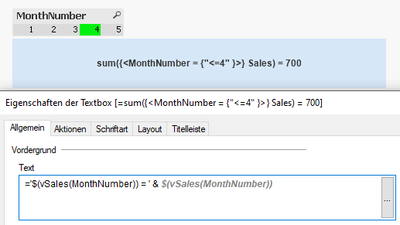Announcements
Product Release Webinar: Qlik Insider airing December 6! REGISTER TODAY!
cancel
Showing results for
Did you mean:Contributor III

## Looking for some help understanding parameters and variables

Hello,

In an effort to get a better understanding of variables, set analysis and \$ expansion, I have a question:

In QlikView, I have this table:

 MonthNumber Sales 1 100 2 300 3 125 4 175 5 400

I also have this variable:

``vSalesSoFar = sum({< MonthNumber = {"<=\$1"} >} ROW_COUNT)``

In a TextBox, when I enter this expression:

``=\$(vSalesSoFar(4))``

I get the result, 700, as expected. It is summing Month Numbers that are less than or equal to 4.

In the table, when I add this expression:

``=\$(vSalesSoFar(MonthNumber))``

I get 0.

My first question is: Why doesn't the expression see the MonthNumber as the parameter?

To check this, I changed the expression to actually enter a number, in the table:

``=\$(vSalesSoFar(4))``

I get 700 on each row, which makes sense to me.

Then I created this variable:

``vMonthPlus = \$1 + 10``

When I entered this expression in the table:

``=\$(vMonthPlus(MonthNumber))``

I get the correct output on each row:

 MonthNumber vMonthPlus 1 10 2 20 3 30 4 40 5 50

My second question is: What is the difference between the two variables that the MonthNumber is recognized in one (vMonthPlus) but not the other (vSalesSoFar)?

Thank you.

1 Solution

Accepted SolutionsPartner - Contributor III

Hi,

as far as I can see, there is an error in your syntax of the ad-hoc variable. Your variable would evaluate to the following syntax:

sum({< MonthNumber = {"<=MonthNumber"} >} Sales)

which just gives "0" as result, since the ad-hoc variable isn't properly declared. If you use the following syntax:

=sum({<MonthNumber = {"<=\$(=max(MonthNumber))" }>} Sales)

you will get the desired result.

To declare that variable in script, with parameters, you have to cut it in half, because QV doesn't allow a variable call within a variable declaration, so it needs to look like this:

let vSales = 'sum({<MonthNumber = {"<=\$' & '(=max(\$1))" }>} Sales)';

And now, when you call the variable in a text box or a chart, it works as desired:

=\$(vSales(MonthNumber))3 RepliesPartner - Contributor III

Hi,

as far as I can see, there is an error in your syntax of the ad-hoc variable. Your variable would evaluate to the following syntax:

sum({< MonthNumber = {"<=MonthNumber"} >} Sales)

which just gives "0" as result, since the ad-hoc variable isn't properly declared. If you use the following syntax:

=sum({<MonthNumber = {"<=\$(=max(MonthNumber))" }>} Sales)

you will get the desired result.

To declare that variable in script, with parameters, you have to cut it in half, because QV doesn't allow a variable call within a variable declaration, so it needs to look like this:

let vSales = 'sum({<MonthNumber = {"<=\$' & '(=max(\$1))" }>} Sales)';

And now, when you call the variable in a text box or a chart, it works as desired:

=\$(vSales(MonthNumber))Contributor

@myccpay wrote:

Hello,

In an effort to get a better understanding of variables, set analysis and \$ expansion, I have a question:

In QlikView, I have this table:

 MonthNumber Sales 1 100 2 300 3 125 4 175 5 400

I also have this variable:

``vSalesSoFar = sum({< MonthNumber = {"<=\$1"} >} ROW_COUNT)``

In a TextBox, when I enter this expression:

``=\$(vSalesSoFar(4))``

I get the result, 700, as expected. It is summing Month Numbers that are less than or equal to 4.

In the table, when I add this expression:

``=\$(vSalesSoFar(MonthNumber))``

I get 0.

My first question is: Why doesn't the expression see the MonthNumber as the parameter?

To check this, I changed the expression to actually enter a number, in the table:

``=\$(vSalesSoFar(4))``

I get 700 on each row, which makes sense to me.

Then I created this variable:

``vMonthPlus = \$1 + 10``

When I entered this expression in the table:

``=\$(vMonthPlus(MonthNumber))``

I get the correct output on each row:

 MonthNumber vMonthPlus 1 10 2 20 3 30 4 40 5 50

My second question is: What is the difference between the two variables that the MonthNumber is recognized in one (vMonthPlus) but not the other (vSalesSoFar)?

Thank you.

Parameters are the things defined by functions as input, arguments are the things passed as parameters.

``````void foo(int bar) { ... }

foo(baz);``````

In this example, bar is a parameter for foo. baz is an argument passed to foo.Contributor III
Author

Hi Lenka_kruse,

I really appreciate your response. Besides getting my expression to work, it was very helpful and caused me to go back and try to get a better understanding of it.

Is the MAX function needed only when using this expression in a table? I tried experminting with the MAX function in and out of the variable and it also seemed to work when MAX was removed. However, if no MonthNumber was selected, it returned 0. I needed to make a MonthNumber number selection to have it return a number.

What is the reason for that?

Thank you so much for the help!Community Browser